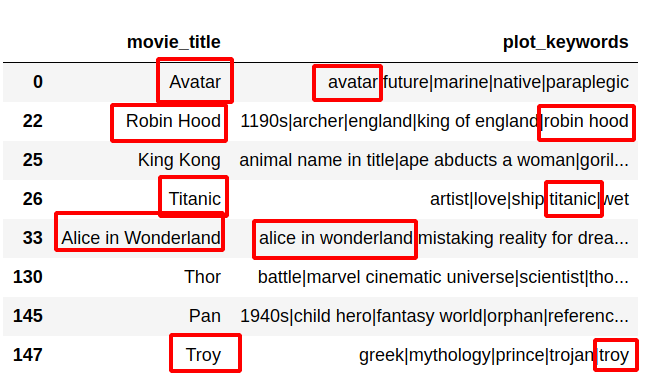In this guide, I'll show you how to find if value in one string or list column is contained in another string column in the same row. In the article are present 3 different ways to achieve the same result.

These examples can be used to find a relationship between two columns in a DataFrame.

Dataset: IMDB 5000 Movie Dataset

## Step 1: Check If String Column Contains Substring of Another with Function

The first solution is the easiest one to understand and work it. It is easy for customization and maintenance.

To start, we will define a function which will be used to perform the check. Then the function will be invoked by using `apply`:

``````def find_value_column(row):
return row.country in row.movie_title

df[df.apply(find_value_column, axis=1)][['movie_title', 'country']]
``````

which will result in:

movie_title country
196 Australia Australia
2504 McFarland, USA USA

What will happen if there are NaN values in one of the columns?

``````def find_value_column(row):
return row.movie_title.lower().strip() in row.plot_keywords

``````

Then error will be raised:

``````TypeError: ("argument of type 'float' is not iterable", 'occurred at index 4')
``````

There is easy solution for this error - convert the column NaN values to empty list values thus:

``````for row in df.loc[df.plot_keywords.isnull(), 'plot_keywords'].index:
df.at[row, 'plot_keywords'] = []
``````

now we get proper results like:## Step 2: Check If Column Contains Another Column with Lambda

The second solution is similar to the first - in terms of performance and how it is working - one but this time we are going to use `lambda`. The advantage of this way is - shortness:

``````df[df.apply(lambda x: x.country in x.movie_title, axis=1)][['movie_title', 'country']]
``````
movie_title country
196 Australia Australia
2504 McFarland, USA USA

A possible disadvantage of this method is the need to know how `apply` and `lambda` works and how to deal with errors if any.

For example this piece of code similar but will result in error like:

``````df.apply(lambda row: df.country in df.movie_title, axis=1)
``````

output:

``````TypeError: ("'Series' objects are mutable, thus they cannot be hashed", 'occurred at index 0')
``````

It may be obvious for some people but a novice will have hard time to understand what is going on.

## Step 3: Fastest Way to Check If One Column Contains Another

This solution is the fastest one. It is short and easy to understand. It includes `zip` on the selected data. In this case data can be used from two different DataFrames.

``````df[[x in x for x in zip(df['country'], df['movie_title'])]][['movie_title', 'country']]
``````
movie_title country
196 Australia Australia
2504 McFarland, USA USA

again if the column contains NaN values they should be filled with default values like:

``````df['country'].fillna('Uknown', inplace=True)
``````

## Step 4: For Loop and df.iterrows() Version

The final solution is the most simple one and it's suitable for beginners. Iterates over the rows one by one and perform the check. This solution is the slowest one:

``````for i, row in df.iterrows():
if row.country in row.movie_title:
print(row.country, row.movie_title)
``````

result:

``````Australia Australia
USA McFarland, USA
``````

## Bonus Step: Check If List Column Contains Substring of Another with Function

Now lets assume that we would like to check if any value from column plot_keywords:

• avatar|future|marine|native|paraplegic
• bomb|espionage|sequel|spy|terrorist

is part of the movie title.

In this case we can:

1. split the string column into a list
2. convert NaN values to empty list
3. perform search for each word in the list against the title
``````df['keywords'] = df.plot_keywords.str.split('|')
``````

Now we have two different options:

Skip the conversion of NaN but check them in the function:

``````def find_value_column(row):
if isinstance(row['keywords'], list):
for keyword in row['keywords']:
return keyword in row.movie_title.lower()
else:
return False

``````

or

Convert all the NaN values:

``````df['keywords'] = df['keywords'].apply(lambda d: d if isinstance(d, list) else [])

def find_value_column(row):
for keyword in row['keywords']:
return keyword in row.movie_title.lower()
return False

``````

results in:## Performance check

Below you can find results of all solutions and compare their speed:

• 10 loops, best of 3: 152 ms per loop
• 10 loops, best of 3: 153 ms per loop
• 1000 loops, best of 3: 1.69 ms per loop
• 1 loop, best of 3: 580 ms per loop

So the one in step 3 - zip one - is the fastest and outperform the others by magnitude. In my everyday work I prefer to use 2 and 3(for high volume data) in most cases and only in some case 1 - when there is complex logic to be implemented.